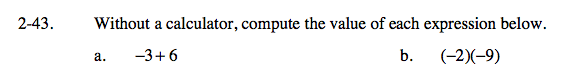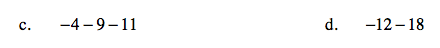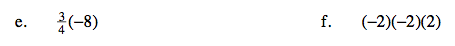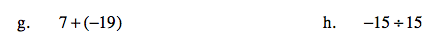### Home > CAAC > Chapter 2 > Lesson 2.1.4 > Problem2-43

2-43.
1. Without a calculator, compute the value of each expression below. Homework Help ✎

1. −3 + 6

2. (−2)(−9)

3. −4 − 9 − 11

4. −12 − 18

5.(−8)

6. (−2)(−2)(2)

7. 7 + (−19)

8. −15 ÷ 153

Keep track of negative signs.Change all the subtraction to addition. −4 + (−9) + (−11)

−24

Follow the steps in part (c).It might be helpful to change −8 to a fraction.

Remember to reduce.

Keep track of negative signs.If the larger number is negative the answer will be negative.

Turn the problem into a fraction.

Any number divided by itself = 1.